Next: Application to General ICA Up: Fast Algorithms for Bayesian Previous: Fast EM-algorithm by Filtering

# FastICA as EM-Algorithm with Filtering of Gaussian Noise

The FastICA algorithm  can be interpreted as performing the above described noise removal. In FastICA the requirement of whitening the sources is also made and therefore Rss=I and (ATA)-1=I. Then, the sources can be found one by one and we can consider a single column aof the mixing matrix A.

To derive the FastICA algorithm from the modified EM-algorithm, it is sufficient to note that the term XGF(s0G)T/M=as0GF(s0G)T/Mis Cfa where Cf is a constant that depends only on the nonlinear function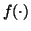. Then the update rule is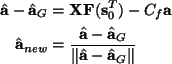which is the FastICA algorithm, where the constant Cf is the expectation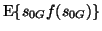.

The choice of fixed nonlinearityis implicitly connected to the distribution of the sources s. The derivation of the EM-algorithm required that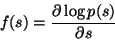However, we see thathas certain degrees of freedom due to taking the difference XF(s0T)-XGF(s0GT). Expanding f polynomially we obtain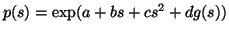where g'(s)=f(s) and g(s) contains all the powers of f higher than two and possibly lower moments too. This representation follows since in the update rule constants and linear terms ofwill cancel out. Therefore they will appear in the distribution p(s) in the exponent with the power raised by one due to integration. Since p(s) must be a probability density, the constant a will be fixed by the requirement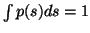. Mean and variance of s will determine the constants b and c, since the sources are required to be zero-mean and whitened (variance is fixed to unity). There is one free parameter d left, which means that there is not only one distribution corresponding tobut a family of p(s). Typically the family includes both super- and sub-Gaussian densities, which is why the samecan be used for both cases.Next: Application to General ICA Up: Fast Algorithms for Bayesian Previous: Fast EM-algorithm by Filtering
Harri Lappalainen
2000-03-09